GFG App
Open AppBrowser
Continue

Transition Table :
Transition function(∂) is a function which maps Q * ∑ into Q . Here ‘Q’ is set of states and ‘∑’ is input of alphabets. To show this transition function we use table called transition table. The table takes two values a state and a symbol and returns next state.

A transition table gives the information about –

1. Rows represent different states.
2. Columns represent input symbols.
3. Entries represent the different next state.
4. The final state is represented by a star or double circle.
5. The start state is always denoted by an small arrow.

Example 1 –
This example shows transition table for NFA(non-deterministic finite automata) .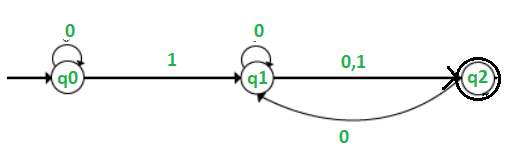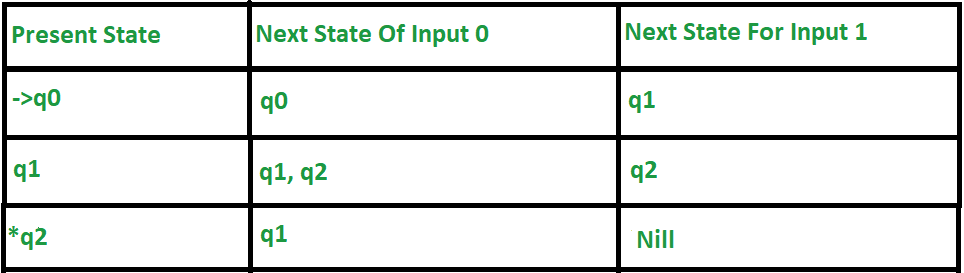Explanation of above table –

1. First column indicates all the present states ,Next for input 0 and 1 respectively.
2. When the present state is q0, for input 0 the next state will become q0. For input 1 the next state is q1.
3. When the present state is q1, for input 0 the next state is q1 or q2, and for 1 input the next state is q2.
4. When the current state is q2 for input 0, the next state will become q1, and for 1 input the next state will become Nil.
5. The small straight arrow on q0 indicates that it is a start state and circle on to q3 indicates that it is a final state.

Example 2 –
This example shows transition table of DFA(deterministic finite automata).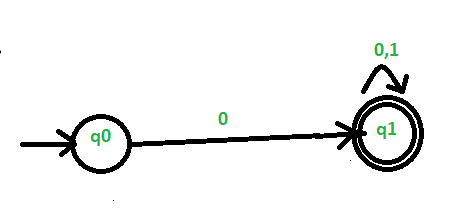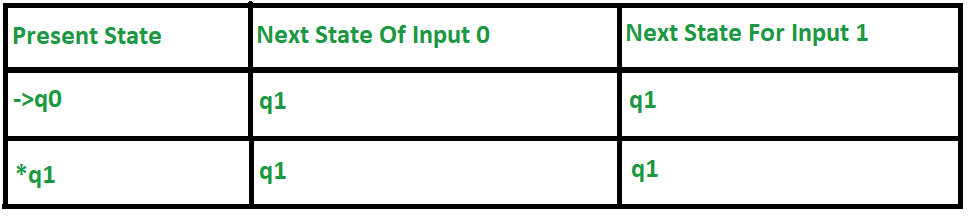Explanation of above table –

1. First column indicates all the present states, Next for input 0 and 1 respectively.
2. When the current state is q0, for input 0 the next state will become q1 and for input as 1 the next state is q1.
3. When the current state is q1, for input 0, the next state will become q1, and on 1 input the next state is q1.
4. The small straight arrow on q0 indicates that it is a start state and circle on to q3 indicates that it is a final state.

Example 3 –
This example shows transition table of DFA(deterministic finite automata)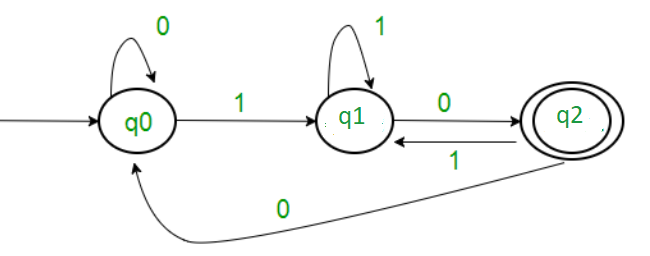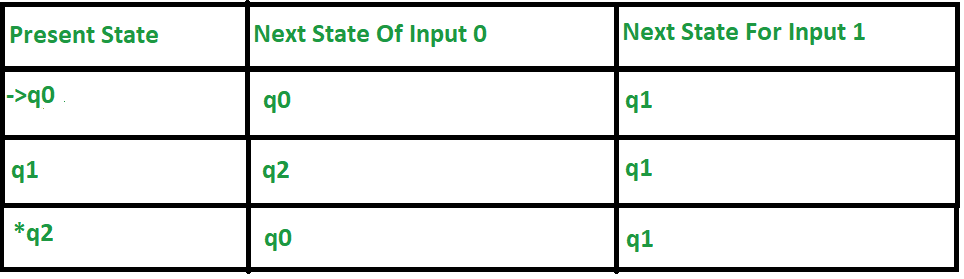Explanation of above table –

1. First column indicates all the present states, Next for input 0 and 1 respectively.
2. When the current/present state is q0, for input 0 the next state will become q0 and for input 1 the next state is q1.
3. When the current state is q1, on input 0, the next state will become q2, and for 1 input the next state is q1.
4. When the current state is q2 for input 0, the next state will become q0, and for 1 input the next state is q1.
5. The small straight arrow on q0 indicates that it is a start state and circle on to q3 indicates that it is a final state.

Example 4 –
This example shows transition table for NFA(non-deterministic finite automata).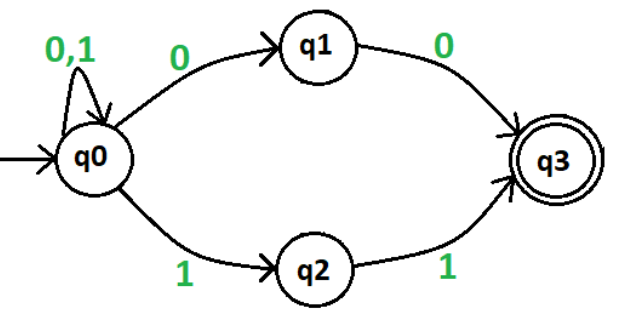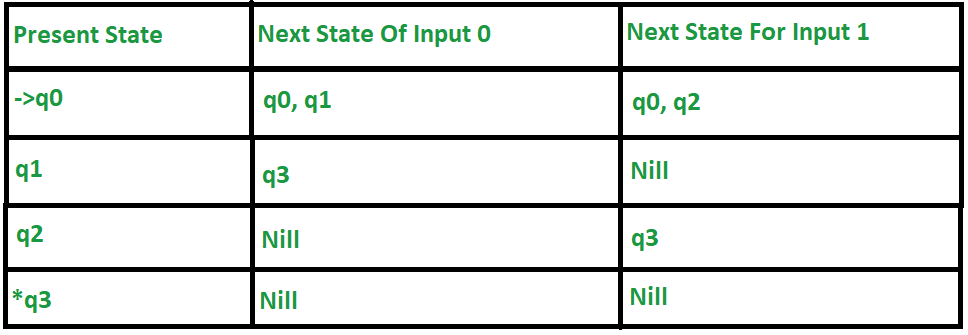Explanation of above table –

1. First column indicates all present states, Next for input 0 and 1 respectively.
2. When the current state is q0, for input 0 next state will become q0 or q1 and for input 1 the next state is q0 or q2.
3. When the current state is q1, for input 0 next state will become q3, and for input 1 the next state is Nil as there is no state for input 1.
4. When the current state is q2 for input 0, next state will become nil as there is no state for input 0, and for 1 input the next state will become q3.
5. When the current state is q3 for input 0, next state will become nil as there is no state for input 0, and for 1 input the next state will also become nil as there is no state for input 1.
6. The small straight arrow on q0 indicates that it is a start state and circle on to q3 indicates that it is a final state.

Note – There can be multiple final states in both DFA and NFA but initial state is unique.

My Personal Notes arrow_drop_up